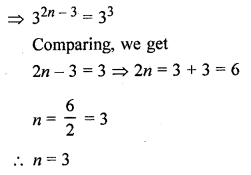## ML Aggarwal Class 8 Solutions for ICSE Maths Chapter 2 Exponents and Powers Ex 2.1

Question 1.
Evaluate:Solution:Question 2.
Simplify: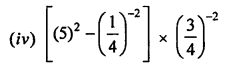Solution: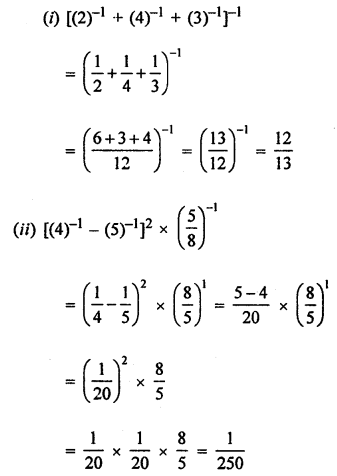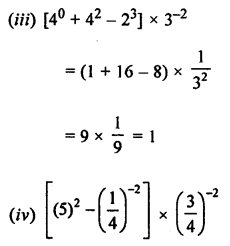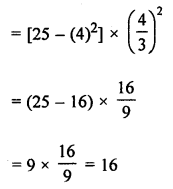Question 3.
Find the multiplicative inverse of the following:Solution: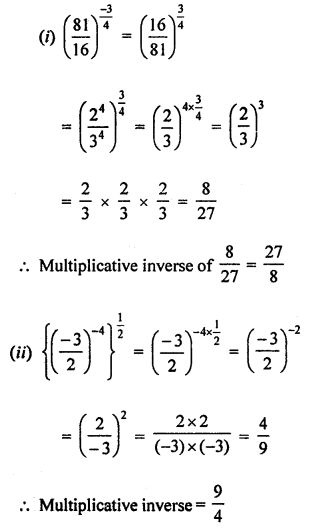Question 4.
(i) Express 16-2 as a power with base 2.
(ii) Express 125-4 as a power with base 5.
Solution: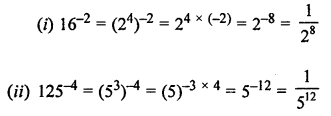Question 5.
Write the following numbers in expanded form using exponents:
(i) 2789.453
(ii) 3007.805
Solution:
(i) 2789.453 = 2 × 103 + 7 × 102 + 8 × 101 + 9 × 100
+ 4 × 10-1 + 5 × 10-2 + 3 × 10-3
(ii) 3007.805 = 3 × 103 + 7 × 100 + 8 × 10-1 + 5 × 10-3

Question 6.
Simplify and write in exponential form with positive exponent:Solution: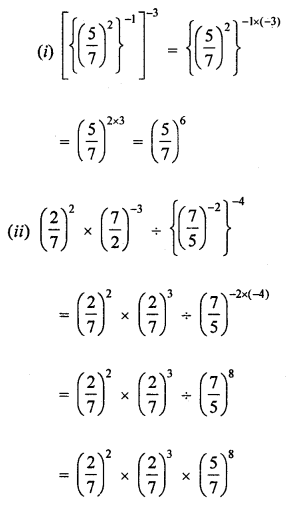Question 7.
Simplify and write the following in exponential form:Solution: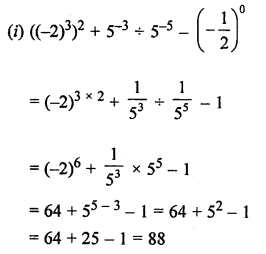Question 8.
Simplify and write in exponential form with negative exponent: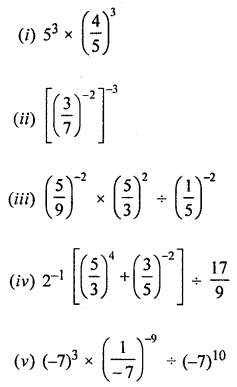Solution: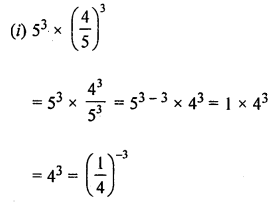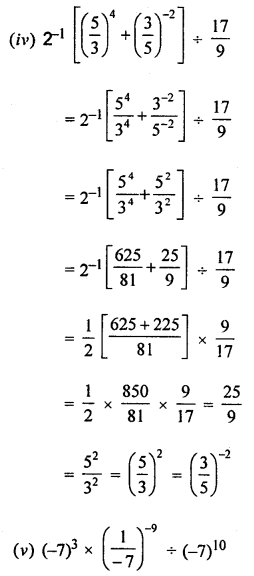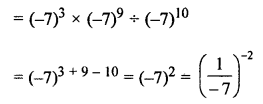Question 9.
Simplify: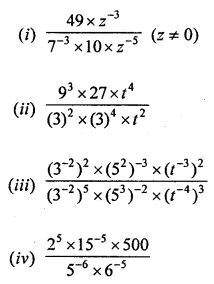Solution:Question 10.
By what number should $$\left( \frac { 3 }{ -2 } \right) ^{ -3 }$$ be divided to get $$\left( \frac { 2 }{ 3 } \right) ^{ 2 }$$ ?
Solution:Question 11.
Find the value of m for which 9m ÷ 3-2 = 94.
Solution:
9m ÷ 3-2 = 94
⇒ (32)m ÷ 3-2 = (32)4
⇒ 32m ÷ 3-2 = 38
⇒ 32m+2 = 38
Comparing, we get
2m + 2 = 8
⇒ 2m = 8 – 2 = 6
⇒ m = $$\frac { 6 }{ 2 }$$ = 3

Question 12.Solution:Question 13.Solution: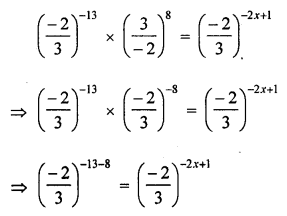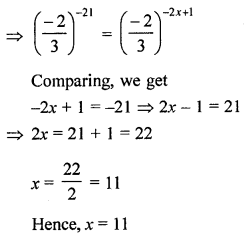Question 14.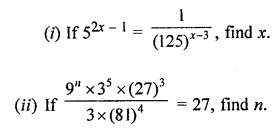Solution: